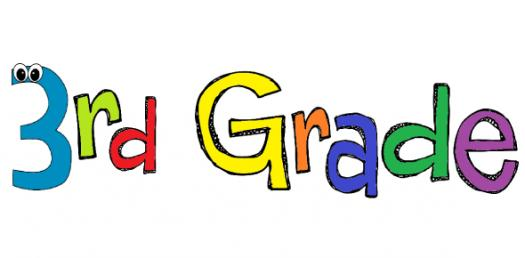# 3rd Grade Math Test On Basic Multiplication! Quiz

20 Questions | Total Attempts: 212SettingsWelcome to this 3rd Grade Math Test on Basic Multiplication Quiz. It is designed to be a work out for students struggling to solve these types of math problems. If you are one of them all you need to do is give it a shot and see how well you will do. All the best as you tackle it and coma back for more.

• 1.
3 X 7 =[Blank]
• 2.
4 X 8 =[Blank]
• 3.
5 X 8 =[Blank]
• 4.
6 X 4 =[Blank]
• 5.
7 X 7 =[Blank]
• 6.
9 X 2 =[Blank]
• 7.
5 X 10 =[Blank]
• 8.
9 X 3 = [Blank]
• 9.
8 X 6 = [Blank]
• 10.
3 X 5 =[Blank]
• 11.
9 X 4 = 36
• A.

True

• B.

False

• 12.
6 X6 = 32
• A.

True

• B.

False

• 13.
5 X 7 = 35
• A.

True

• B.

False

• 14.
8 X 3 = 24
• A.

True

• B.

False

• 15.
2 X7 = 15
• A.

True

• B.

False

• 16.
3 X 7 =
• A.

24

• B.

21

• C.

18

• D.

14

• 17.
6 X 7 =
• A.

42

• B.

41

• C.

54

• D.

46

• 18.
8 X 5 =
• A.

45

• B.

40

• C.

35

• D.

30

• 19.
9 X 2 =
• A.

20

• B.

19

• C.

18

• D.

15

• 20.
2 X 7 =
• A.

15

• B.

16

• C.

20

• D.

14

Related TopicsBack to top